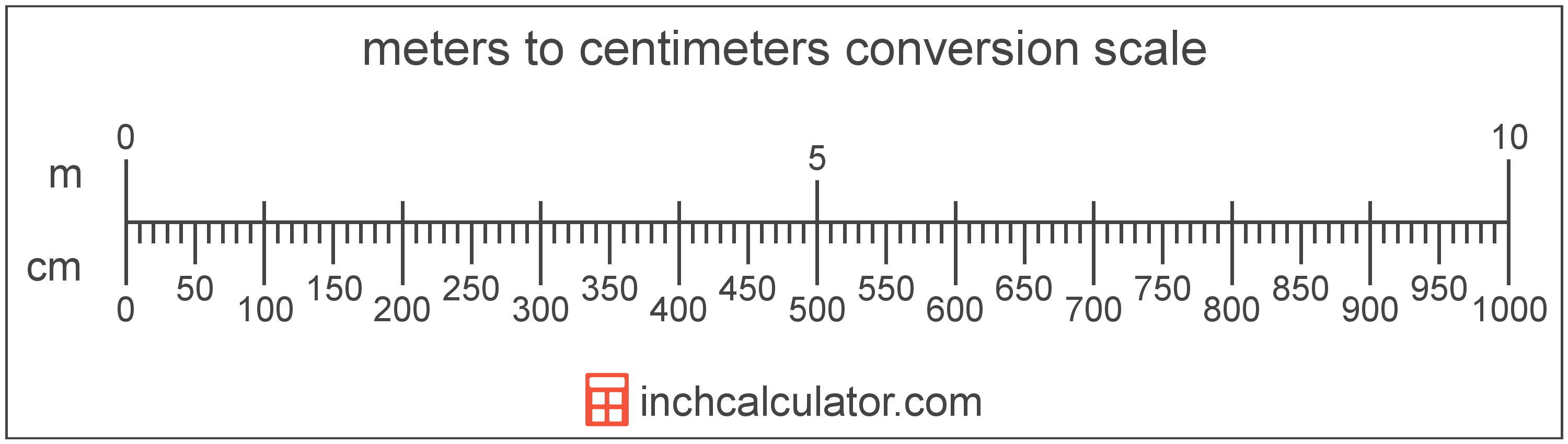# Centimeters to Meters Conversion

Enter the length in centimeters below to get the value converted to meters.

Results in Meters:1 cm = 0.01 m

## How to Convert Centimeters to MetersTo convert a centimeter measurement to a meter measurement, divide the length by the conversion ratio.

Since one meter is equal to 100 centimeters, you can use this simple formula to convert:

meters = centimeters ÷ 100

The length in meters is equal to the centimeters divided by 100.

For example, here's how to convert 500 centimeters to meters using the formula above.
500 cm = (500 ÷ 100) = 5 m

Our inch fraction calculator can add centimeters and meters together, and it also automatically converts the results to US customary, imperial, and SI metric values.

## Centimeters

One centimeter is equal to one-hundredth (1/100) of a meter, which is defined as the distance light travels in a vacuum in a 1/299,792,458 second time interval.

The centimeter, or centimetre, is a multiple of the meter, which is the SI base unit for length. In the metric system, "centi" is the prefix for 10-2. Centimeters can be abbreviated as cm; for example, 1 centimeter can be written as 1 cm.

Metric rulers typically have 30 cm, which are represented by 30 large tick marks. To get a rough idea of the actual length of a centimeter, a standard pencil is just about 1 cm thick.

## Meters

The meter is defined as the length of the path traveled by light in vacuum during a time interval with a duration of 1/299,792,458 of a second, according to the most recent 2019 definition. One meter is equal to 100 centimeters or 39.37 inches.

The meter, or metre, is the SI base unit for length in the metric system. Meters can be abbreviated as m; for example, 1 meter can be written as 1 m.

We recommend using a ruler or tape measure for measuring length, which can be found at a local retailer or home center. Rulers are available in imperial, metric, or combination with both values, so make sure you get the correct type for your needs.

Need a ruler? Try our free downloadable and printable rulers, which include both imperial and metric measurements.

## Centimeter to Meter Conversion Table

Centimeter measurements converted to meters
Centimeters Meters
1 cm 0.01 m
2 cm 0.02 m
3 cm 0.03 m
4 cm 0.04 m
5 cm 0.05 m
6 cm 0.06 m
7 cm 0.07 m
8 cm 0.08 m
9 cm 0.09 m
10 cm 0.1 m
20 cm 0.2 m
30 cm 0.3 m
40 cm 0.4 m
50 cm 0.5 m
60 cm 0.6 m
70 cm 0.7 m
80 cm 0.8 m
90 cm 0.9 m
100 cm 1 m

## References

1. International Bureau of Weights and Measures, The International System of Units, 9th Edition, 2019, https://www.bipm.org/documents/20126/41483022/SI-Brochure-9-EN.pdf